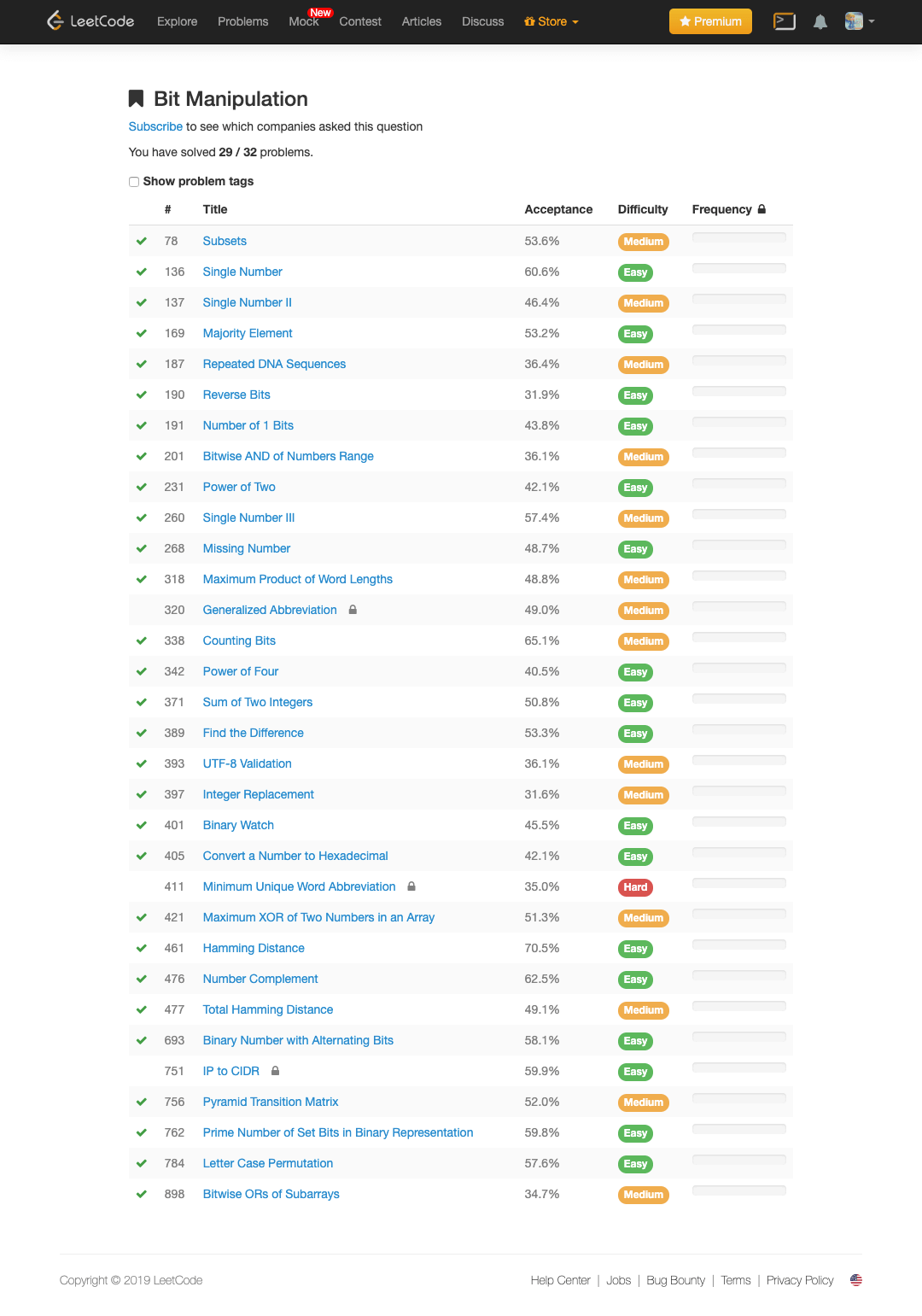## Bit Manipulation 的 Tips:

• 异或的特性。第 136 题，第 268 题，第 389 题，第 421 题，
``````x ^ 0 = x
x ^ 11111……1111 = ~x
x ^ (~x) = 11111……1111
x ^ x = 0
a ^ b = c  => a ^ c = b  => b ^ c = a (交换律)
a ^ b ^ c = a ^ (b ^ c) = (a ^ b）^ c (结合律)
``````
• 构造特殊 Mask，将特殊位置放 0 或 1。
``````1. 将 x 最右边的 n 位清零， x & ( ~0 << n )
2. 获取 x 的第 n 位值(0 或者 1)，(x >> n) & 1
3. 获取 x 的第 n 位的幂值，x & (1 << (n - 1))
4. 仅将第 n 位置为 1，x | (1 << n)
5. 仅将第 n 位置为 0，x & (~(1 << n))
6. 将 x 最高位至第 n 位(含)清零，x & ((1 << n) - 1)
7. 将第 n 位至第 0 位(含)清零，x & (~((1 << (n + 1)) - 1)）
``````
• 有特殊意义的 & 位操作运算。第 260 题，第 201 题，第 318 题，第 371 题，第 397 题，第 461 题，第 693 题，
``````X & 1 == 1 判断是否是奇数(偶数)
X & = (X - 1) 将最低位(LSB)的 1 清零
X & -X 得到最低位(LSB)的 1
X & ~X = 0
``````
Title Solution Difficulty Time Space 收藏
78. Subsets Go Medium O(n^2) O(n) ❤️
136. Single Number Go Easy O(n) O(1)
137. Single Number II Go Medium O(n) O(1)
169. Majority Element Go Easy O(n) O(1) ❤️
187. Repeated DNA Sequences Go Medium O(n) O(1)
190. Reverse Bits Go Easy O(n) O(1)
191. Number of 1 Bits Go Easy O(n) O(1)
201. Bitwise AND of Numbers Range Go Medium O(n) O(1) ❤️
231. Power of Two Go Easy O(1) O(1)
260. Single Number III Go Medium O(n) O(1)
268. Missing Number Go Easy O(n) O(1)
318. Maximum Product of Word Lengths Go Medium O(n) O(1)
338. Counting Bits Go Medium O(n) O(n)
342. Power of Four Go Easy O(n) O(1)
371. Sum of Two Integers Go Easy O(n) O(1)
389. Find the Difference Go Easy O(n) O(1)
393. UTF-8 Validation Go Medium O(n) O(1)
397. Integer Replacement Go Medium O(n) O(1)
401. Binary Watch Go Easy O(1) O(1)
405. Convert a Number to Hexadecimal Go Easy O(n) O(1)
421. Maximum XOR of Two Numbers in an Array Go Medium O(n) O(1) ❤️
461. Hamming Distance Go Easy O(n) O(1)
476. Number Complement Go Easy O(n) O(1)
477. Total Hamming Distance Go Medium O(n) O(1)
693. Binary Number with Alternating Bits Go Easy O(n) O(1) ❤️
756. Pyramid Transition Matrix Go Medium O(n log n) O(n)
762. Prime Number of Set Bits in Binary Representation Go Easy O(n) O(1)
784. Letter Case Permutation Go Easy O(n) O(1)
898. Bitwise ORs of Subarrays Go Easy O(n) O(1)
Previous Post

Next Post

### LeetCode 分类刷题 —— Union Find

You've successfully subscribed to Halfrost's Field | 冰霜之地
Great! Next, complete checkout for full access to Halfrost's Field | 冰霜之地
Welcome back! You've successfully signed in.